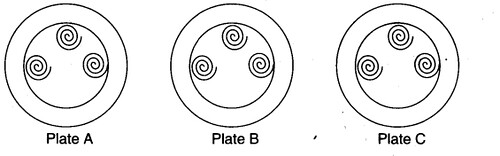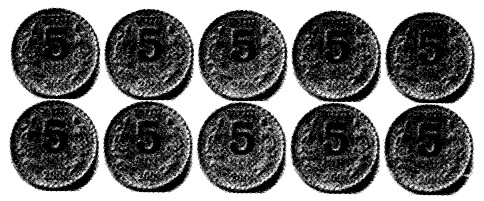# NCERT Solutions for class 3 Mathematics Chapter-12 Can We Share ?

We provided NCERT Solutions for Class 3 Maths Chapter 12 Can We Share ? is prepared by the best teachers across India. These NCERT 3rd Class Maths Chapter 12 Can We Share ? Solution includes detailed answers of all the questions in Chapter 12 Can We Share ? provided in NCERT Book which is prescribed for Class 3 in CBSE school Books. This will help students to understand basic concepts better.

## CBSE NCERT 3rd Class Maths Chapter 12 Can We Share ? Solutions

 Organisation National Council of Educational Research and Training Class Name 3rd Class Subject Name Maths Chapter Name Chapter 12 Content name Can We Share ? Category Name CBSE NCERT Solutions Official Website http://ncert.nic.in/

### NCERT 3rd Class Maths Chapter 12 Can We Share ? Solution is given below

Share the Grains
1.Mummy bird brings 12 grains.
How to distribute equally?
Mummy bird starts by giving 1 grain to each baby. Then mummy bird gives one more grain to each baby. Each baby has got 2 grains now. How many grains are
Ans. There are 4 baby birds and each baby bird gets 2 grains.
So, number of grains with baby birds = 2×4 = 8
So, number of grains left = 12 – 8 = 4
She puts one more grain in each baby’s mouth. All the grains are finished.
12 grains have been divided among 4 baby birds. Each baby has got 3 grains. 12÷4 = 3

Try These Now
Gopu has 3 plates of jalebis.
Each plate has a different number of jalebis.Now draw the jalebis on the plates below, so that each plate has the same number of jalebis.1.How many jalebis are there altogether?
Ans. 1 + 5 + 3 = 9 jalebis.

2.How many jalebis are there in each plate?
Ans. 3 jalebis.

3.Discuss in the class how you found the answer.
Ans. Total number of jalebis = 1 + 5 + 3 = 9
Total number of jalebis is divided into 3 equal parts:
9÷3 = 3
So, each plate gets 3 jalebis.

Sharing Them Equally?
Here are six bananas.
Here are three monkeys.
If they share the bananas equally, each monkey will get 2 bananas.
6 bananas divided into 3 equal parts = 2 bananas each 6 + 3 = 2
If there are six bananas and two monkeys,
Each monkey will get three bananas.
Six bananas + 2 = 3 bananas each.
6 ÷2 = 3

1.If there are 60 bananas and two monkeys, how many will each monkey get?
Ans. 60 ÷ 2 = 30 bananas
So, each monkey will get 30 bananas.

2.What if there are 600 bananas and two monkeys?
Ans. 600 ÷ 2 = 300 bananas
So, each monkey will get 300 bananas.Five friends found 10 five-rupee coins on the ground. They shared them equally. Each friend got ten rupees.
50 ÷ 5 = 10
If there are 16 ten-rupee notes and four friends to share, then 16 ÷ 4 = 4 ten-rupee notes and 4 x 10 = 40. So, each friend gets 4 x 10 = 40
rupees.

1.Five friends found ? 100. If they share it equally, how much will each get?
Ans.100÷ 5 = 20.

2. Hari  Prasad has 30 metres of rope.
He distributes it equally among his three children. Each child gets———–metres of rope.
Ans. 30÷3 = 10 metres.

3.If there is 36 metres of rope, how much of rope will each child get?
Ans. 36 ÷ 3 = 12 metres.

4.If there is 60 metres of rope, how much will each child get?
Ans. 60 ÷ 3 = 20 metres.

How Many Shelves?
1.I have 20 books. I can keep 5 books in one shelf, so how many shelves do I need in my almirah?
Ans. 20÷5 = 4 shelves.

Practice Time
1. Minku puts her 15 laddoos equally into 5 boxes.
(i)How many laddoos will there be in each box?
Ans. 15 ÷ 5 = 3 laddoos in each box.
(ii)If she uses only 3 boxes, how many laddoos will there be in each box?
Ans. 15 ÷ 3 = 5 laddoos in each box.

2.Share 25 bananas among 5 monkeys. How many bananas for each monkey?
Ans. 25 ÷5 = 5 bananas for each monkey.

3.Share 12 balloons among 3 boys. How many balloons for each boy?
Ans. 12÷ 3 = 4 balloons for each boy.

4.There are 21 candles. Put them equally in 3 boxes. How many candles are there in each box?
Ans. 21 ÷ 3 = 7 candles in each box.

5.There are 18 socks. How many girls can wear these socks?
Ans. One girl wears 2 socks.
So, 18÷ 2 = 9 girls can wear these socks.
Raj has 36 minutes to make rotis. One roti takes 3 minutes.

6.How many rotis can he make in this time?
Ans. 36 ÷ 3 = 12
So, Raj can make 12 rotis in this time.

7.There are 24 foot marks of goats. So, how many goats are there?
Ans. 1 goat will make 4 foot marks , So, 24 -r 4 = 6 goats are there.

8.Some girls are playing a game with both their hands. The girls,“who are playing, ! have 60 fingers; altogether. How many girls are playing this game?
Ans. Each girl has 10 fingers.
So, 60 ÷10 = 6 girls are playing this game.

9.Lakshmi has 27 kg potatoes to sell. Three men came and bought equal amounts—————of potatoes. Each man bought kg of potatoes.
Ans. 27 ÷ 3 = 9 kg of potatoes.

Jumpy Animals
1.In how many jumps will the frog reach 30?
Ans. 30÷2 = 15.

2.In how many jumps will the squirrel reach 27?
Ans. 27÷ 3 = 9.

3.Which number will the kangaroo reach in two jumps?
Ans. 30 x 2 = 60

4.Who all will meet at the number 15?
Ans. Squirrel, rabbit, horse.
Explanation: One jump of squirrel is 3 steps and 15 is divisible by 3 One jump of rabbit is 5 steps and 15 is divisible by 5 One jump of horse is 15 steps.
So, all these three animals can meet at the number 15.

5.Will the rabbit ever be at the number 18?
Ans. No.
Explanation: One jump of rabbit is 5 steps and 18 is not divisible by 5. So, rabbit can never reach number 18.

6.How many jumps of the rabbit equal one jump of the horse?
Ans. One jump of horse = 15 steps One jump of rabbit = 5 steps
So, 15÷ 5 = 3 jumps of rabbit equal one jump of the horse.

7.How’ many jumps of the horse equal two jumps of the kangaroo?
Ans. One jump of kangaroo = 30 steps
So, two jumps of kangaroo = 30 x 2 = 60 steps One jump of horse = 15 steps
So, 60 ÷15 = 4 jumps of horse equal two jumps of kangaroo.

8.Which is the smallest number where the frog and the squirrel will meet?
Ans. One jump of frog = 2 steps
One jump of squirrel = 3 steps And, 2×3 = 6
So; the smallest number where the frog and the squirrel will meet is 6.